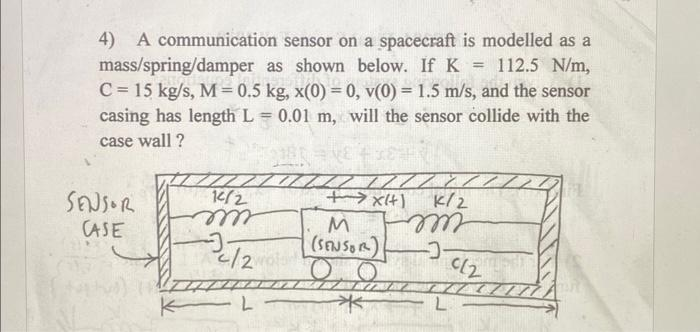Home / Expert Answers / Mechanical Engineering / 4-a-communication-sensor-on-a-spacecraft-is-modelled-as-a-mass-spring-damper-as-shown-below-if-k-pa705

# (Solved): 4) A communication sensor on a spacecraft is modelled as a mass/spring/damper as shown below. If K= ...4) A communication sensor on a spacecraft is modelled as a mass/spring/damper as shown below. If , , and the sensor casing has length , will the sensor collide with the case wall?

We have an Answer from Expert

To determine whether the communication sensor on the spacecraft will collide with the case wall, we need to analyze the motion of the mass.

The equation of motion for the mass can be derived using Newton's second law:
Mx''(t) + Cx'(t) + Kx(t) = 0
where x(t) is the displacement of the mass from its equilibrium position at time t, x'(t) is the velocity of the mass at time t, and x''(t) is the acceleration of the mass at time t.
Substituting the given values for M, C, K, x(0), and v(0), we can solve for x(t) using the characteristic equation:
Ms^2 + Cs + K = 0
We have an Answer from Expert